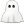# typeracer

Pit Stop

 Racer Rudy (rudy_yt) Race Number 69 Date Mon, 13 Sep 2021 12:57:39 +0000 Universe repeat Speed 51 WPMTry to beat? Accuracy 97.2% Rank 2nd place (out of 2)ask ask ask ask ask ask ask ask ask ask ask ask ask ask ask ask ask ask ask ask ask ask ask ask ask ask ask ask ask ask ask ask ask ask — Repeating Words(other) by TypeRacer (see stats)